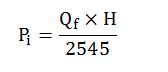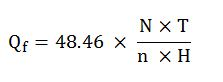Rig Engine Power Consumption and Engine Efficiency

In this article, we will focus on the rig engine power consumption and overall engine efficiency and there are few examples for you to get more understand on how to use the formulas as well.Input Power of Engine

The formula is listed below;Where;

Pi = input power in horse power (hp)

H = fuel heating value in BTU/lb

Qf = fuel consumption rate in lbm/hr

Fuel Consumption

The formula is listed below;